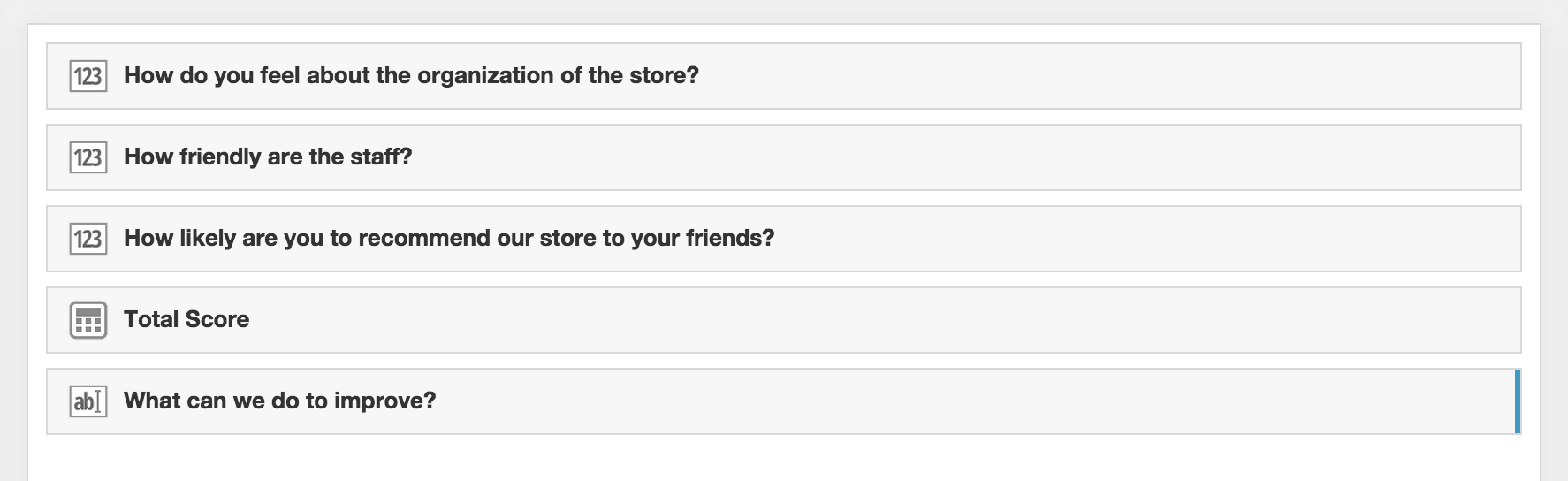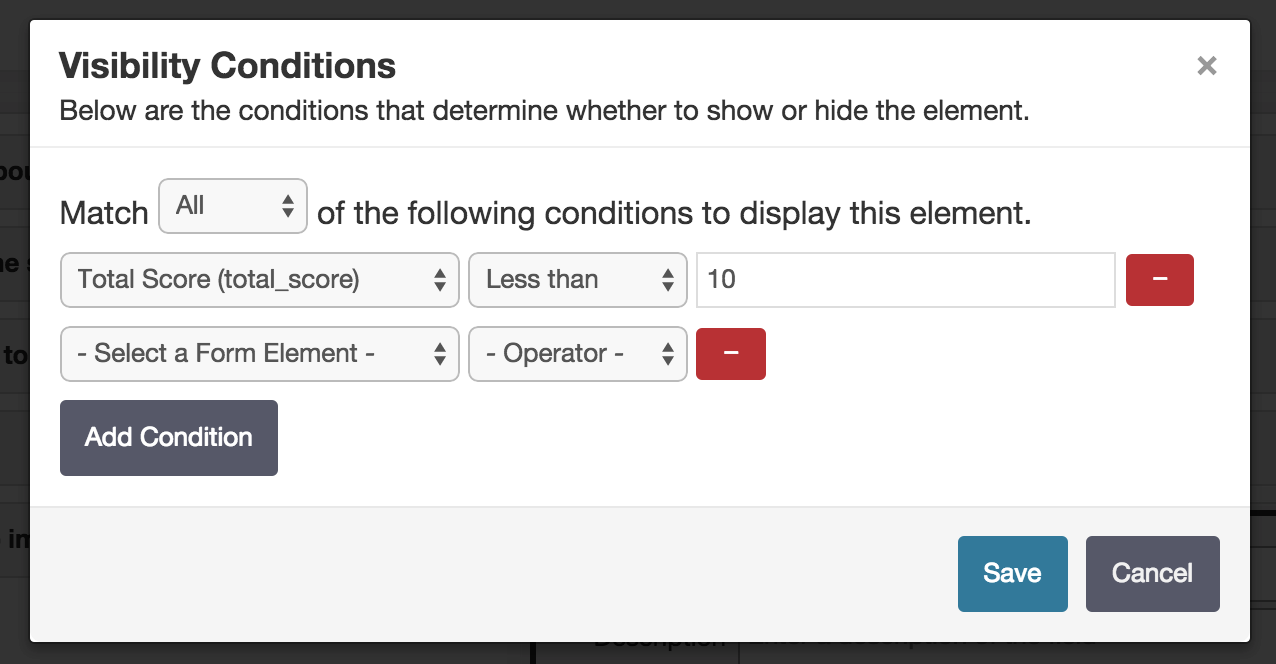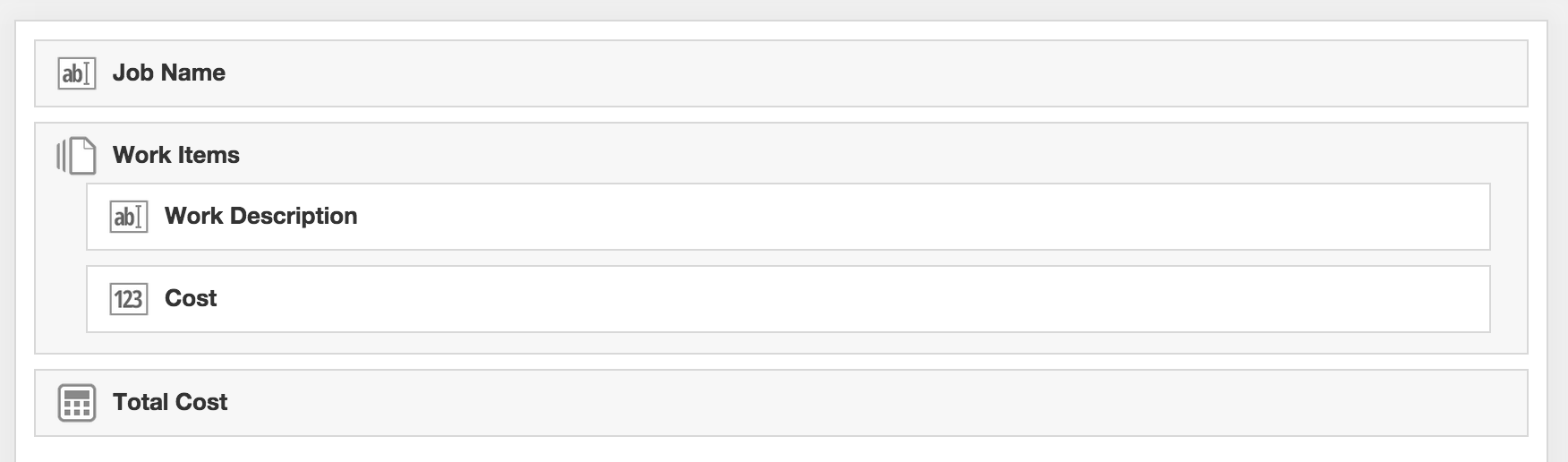5 Tips for Using Calculation Fields

# 5 Tips for Using Calculation Fields

Fulcrum’s calculation fields enable some powerful collection capabilities. There can be many ways to configure and set them up, so this post provides some tips and tricks to help you get the most out of your data collection workflow.

### Complex Condition Logic

One of the most powerful features of calculation fields is using the calculated values in visibility conditions in other fields on the form. This is best depicted with an example. Suppose you are doing a customer satisfaction survey and there are three questions asking a person to rate the customer service on a scale of one to five. If the sum of the ratings is less than ten, you want to ask another question about how the service could be improved. This can be accomplished using a combination of calculation fields and condition logic.

Here’s a screenshot of the survey so you can get a sense of what it looks like:First, we need a calculation field to store the total score. The total score field will function as a ‘variable’ we can use in a condition. You can also hide calculation fields if you don’t want the result to be visible. This is useful if the calculated value is only being used in a condition. In this example, we’ll just leave the total score visible to the user. The expression for the total score is:

``````\$store_organization + \$staff_friendliness + \$recommendation_likelihood
``````

After setting up the calculation field, we can now configure the visibility condition on the “What can we do to improve?” followup field. If you’re already familiar with visibility conditions, conditions on calculation fields work exactly the same as conditions on any other field type.Now, when the score is less than 10, the additional field will be displayed to capture the customer’s feedback. You can even chain this technique together with multiple sets of fields, calculations and conditions to form even more sophisticated workflows.

### Repeatable Data

It’s also possible to aggregate data across entries in repeatable fields. For example, in a job estimation form, we could have a ‘Work Items’ repeatable field that captures the line items of work to be completed. Within each work item, there’s a numeric field to capture the cost of the work. In the parent section of the survey, there’s a calculation field that displays the total cost to complete all the work items.

Below is an example showing the app structure:The expression for the ‘Total Cost’ calculation field is:

``````REPEATABLESUM(\$work_items, 'cost')
``````

In the expression above, the `REPEATABLESUM` function is saying, “Sum up all of the values from the ‘cost’ field inside the work items repeatable.” This function is specifically designed for summing numeric fields inside of repeatables.

As new work items are entered, a running total is kept on the parent record. This example is intentionally simple to demonstrate the functional building blocks. In practice, this can be combined with other calculation fields and numeric fields inside the repeatable to perform composite calculations. In the example above, the ‘cost’ field inside the repeatable section could itself be a calculation field that is derived from other numeric fields such as an hourly rate and number of hours entered on each work item.

You can see a more complete example in the Job Estimation App from the app gallery.

### It’s Not Just Numbers

Another neat feature of calculation fields is using them to ‘calculate’ text. Using this technique, you can display dynamic text data to the user. An example is concatenating several values together to form a summary of the data.

Below is an example expression for a summary field at the bottom of the job estimation app.

``````if (NOT(ISBLANK(\$total_cost)) && NOT(ISBLANK(\$job))) {
SETRESULT("The total cost of " + \$job + " is " + DOLLAR(\$total_cost) + ".")
}
else {
SETRESULT("")
}
``````

This expression is slightly more complex than the previous examples. Let’s review the parts so we can understand what’s happening. It’s best practice to check for the existence of data before trying to use it like this. In Fulcrum, any field can be empty. In our example, we don’t want it to display “The total cost of is “ when there’s no data in the fields yet. To accomplish this in our expression, we check for the existence of the values before building the text string. In this expression, we’re using the built-in `NOT` and `ISBLANK` functions to check for the existence of data in the fields. There are multiple ways to accomplish this in JavaScript, but for the purposes of this example we’re using the built-in functions so it handles some of the idiosyncrasies of JavaScript. Also in this expression, we’re using the `SETRESULT` function. This function can be used to set the result of the current expression. For simple one-line expressions like the previous examples, `SETRESULT` isn’t necessary. But when expressions have complex nesting and multiple branches of execution, we can use this function to clearly indicate what the final resulting value should be.

### Use the Display Format

We highly recommend using the proper display format for calculation fields. The most important tip is for numeric calculations. In general, avoid building text strings for numeric data. We spent a lot of time making it very easy to display formatted numeric data while keeping the underlying raw data in a machine-readable format. For example, there’s rarely a reason to build currency text values. The only time you would normally need to build currency values manually is if you’re returning summary text like the example above. If you’re returning a single value, it’s best to avoid using the `DOLLAR` function.

When displaying a currency value, instead of:

``````DOLLAR(\$item_cost * \$item_quantity)
``````

Set the display format to currency (and set the currency denomination - USD, euro, etc.) and use this:

``````\$item_cost * \$item_quantity
``````

We’ve removed the `DOLLAR` function from the expression and let the display formatting handle it. This will keep the raw data as a number and has several major benefits. The biggest benefit is being able to use the calculation result in another numeric calculation. The result of the new calculation is still a number. If we had made the expression include the `DOLLAR` function, we can’t do math on that value since it’s now a formatted text value. In addition to preserving numericality for chained calculations, you can also export your data to Excel or other formats and retain the raw number value. This allows you to do further interesting things with the data as numbers. There are valid cases to include formatting in the expression, but we strongly recommend against it when it’s possible to use the display formats.

### Combining It All

Where calculation fields get really interesting is when you combine all of these techniques together. We designed calculation fields so they’re a natural extension of the platform and slot in nicely with the existing powerful features like visibility and requirement rules. You can read more about calculation fields in our documentation.

If you have any feedback about how you’re using calculation fields in your apps, we’d love to hear about it, so send us a message at support@fulcrumapp.com.

Calculator by Stephen JB Thomas is used under a CC BY license.